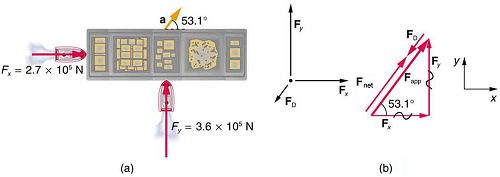$$\require{cancel}$$

# 8.4: The Newtonian Model Summary

•• Contributed by Physics 7
• Physics at UC Davis

We summarize here the main ideas of the Newtonian model/approach by listing the (1) constructs, i.e., the “things” or ideas that are get “used” in the model, (2) the relationships–in mathematical or sentence form–that connect the constructs in meaningful ways, and (3) the ways of representing the relationships. Developing a deep and rich understanding of the relationships in a model/approach comes slowly. It is absolutely not something you can simply memorize. This understanding comes only with repeated hard mental effort over a period of time. A good test you can use to see if you are “getting it” is whether you can tell a full story about each of the relationships. It is the meaning behind the equations, behind the simple sentence relationships, that is important for you to acquire. With this kind of understanding, you can apply a model/approach to the analysis of phenomena you have not thought about before. You can reason with the model.

Example: Drag Force on a Barge

Suppose two tugboats push on a barge at different angles, as shown in Figure. The first tugboat exerts a force of $$2.7 \times 10^5 \space N$$ in the x-direction, and the second tugboat exerts a force of $$3.6 \times 10^5 \space N$$ in the y-direction.Figure 8.4.1. (a) A view from above of two tugboats pushing on a barge. (b) The free-body diagram for the ship contains only forces acting in the plane of the water. It omits the two vertical forces—the weight of the barge and the buoyant force of the water supporting it cancel and are not shown. Since the applied forces are perpendicular, the x- and y-axes are in the same direction as $$F_x$$ and $$F_y$$ The problem quickly becomes a one-dimensional problem along the direction of $$F_{app}$$, since friction is in the direction opposite to $$F_{app}$$.

If the mass of the barge is $$5.0 \times 10^6 \space kg$$ and its acceleration is observed to be $$7.5 \times 10^{-2} \space m/s^2$$ in the direction shown, what is the drag force of the water on the barge resisting the motion? (Note: drag force is a frictional force exerted by fluids, such as air or water. The drag force opposes the motion of the object.)

Strategy

The directions and magnitudes of acceleration and the applied forces are given in Figure (a). We will define the total force of the tugboats on the barge as $$F_{app}$$ so that:$F_{app} = F_x + F_y$

Since the barge is flat bottomed, the drag of the water $$F_D$$ will be in the direction opposite to $$F_{app}$$ as shown in the free-body diagram in Figure (b). The system of interest here is the barge, since the forces on it are given as well as its acceleration. Our strategy is to find the magnitude and direction of the net applied force $$F_{app}$$, and then apply Newton’s second law to solve for the drag force $$F_D$$.

Solution

Since $$F_x$$ and $$F_y$$ are perpendicular, the magnitude and direction of $$F_{app}$$ are easily found. First, the resultant magnitude is given by the Pythagorean theorem:

$F_{app} = \sqrt{F_x^2 + F_y^2}$

$F_{app} = \sqrt{(2.7 \times 10^5 \space N)^2 + (3.6 \times 10^5 \space N)^2} = 4.5 \times 10^5 \space N.$

The angle is given by $\theta = tan^{-1} \left(\dfrac{F_y}{F_x} \right)$

$\theta = tan^{-1} \left( \dfrac{3.6 \times 10^5 \space N}{2.7 \times 10^5 \space N} \right) = 53^o,$

which we know, because of Newton’s first law, is the same direction as the acceleration. $$F_D$$ is in the opposite direction of $$F_{app}$$, since it acts to slow down the acceleration. Therefore, the net external force is in the same direction as $$F_{app}$$, but its magnitude is slightly less than $$F_{app}$$. The problem is now one-dimensional. From Figure (b) we can see that

$F_{net} = F_{app} - F_D.$ But Newton’s second law states that $F_{net} = ma$

Thus, $F_{app} - F_D = ma$

This can be solved for the magnitude of the drag force of the water $$F_D$$ in terms of known quantities: $F_D = F_{app} - ma$ Substituting known values gives $F_D = (4.5 \times 10^5 \space N) - (5.0 \times 10^6 \space kg)(7.5 \times 10^{-2} \space m/s^2) = 7.5 \times 10^4 \space N$

The direction of $$F_D$$ has already been determined to be in the direction opposite to $$F_{app}$$ or at an angle of $$53^o$$ south of west.

Discussion

The numbers used in this example are reasonable for a moderately large barge. It is certainly difficult to obtain larger accelerations with tugboats, and small speeds are desirable to avoid running the barge into the docks. Drag is relatively small for a well-designed hull at low speeds, consistent with the answer to this example, where $$F_D$$ is less than 1/600th of the weight of the ship.

Listed here are the major, most important constructs, relationships, and representations of the Newtonian model.

# Constructs

Force as the agent of interaction between two objects

$$F_{A\:on\:B}$$

net force $$\Sigma F$$(net Torque $$\Sigma\tau$$ )

acceleration, $$a$$ angular acceleration, $$\alpha$$

mass rotational inertia

components

# Relationships

Newton's 3rd law:

$F_{A\:on\:B}=-F_{B\:on\:A}$

$J_{A\:on\:B}=-J_{B\:on\:A}$

Newton's 1st law:

$If\: \Sigma F = 0,\: then\: \Delta v = 0$

Newton's 2nd law:

$\Sigma F= dp/dt \enspace \Sigma F= ma \enspace \Sigma\tau = dL/dt \enspace \Sigma\tau = I\alpha$

Special Case of Constant Acceleration

$x(t) = x_0 + v_{x0} t + \frac{1}{2} a_{x0} t^2 ,$

$v_x(t) = v_{x0} + a_{x0}t,$

$a_x(t)= a_{x0}$

Rotation

$\frac{ds}{dt} = v_{tangential}= r\frac{d\theta}{dt}$

$\omega = \frac{d\theta}{dt} ~~~~~~~~~~\enspace v_{tangential}=r\omega$

$\alpha= \frac{d\omega}{dt} ~~~~~~~~~~\enspace a_tangential=r\alpha$

Special Case of circular motion as constant speed

$v_{tangential}=r\omega$

$v_{radial}=0.$

$a_{radial}=-r\omega^2 = -\frac{v^2}{r}$

$a_{tangential} = 0$

$\Sigma F_{radial}=ma_{radial}= -mr\omega^2=-\frac{mv^2}{r}$

Representations

Force Diagrams

Vector(arrow) representation of Newton's laws